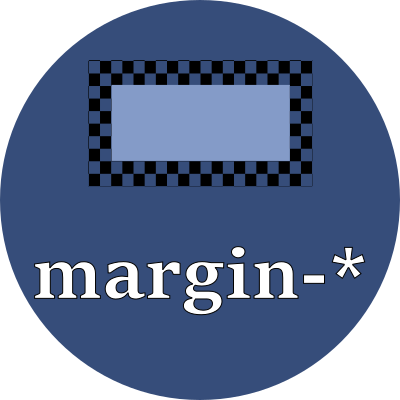# margin-bottomThe white space between this element's bottom border and the top of the next element, which is not filled with the background color.

## Property values

Note that in many cases an element's margin-bottom is compared to the next element's margin-top, and the larger of the two is kept as white-space (not the sum of the two). This is referred to as margin collapsing.

Use any of these font-relative typographic units to set the margin's height:

 em em The `font-size` of the current font rem relative em The `font-size` of the element lh line height The `line-height` of the current font rlh relative line height The `line-height` of the element cap cap height The nominal height of the current font's capital letters ex "x" The height of the current font's "x" glyph ch "0" The advance measure (the width) of the current font's "0" glyph ic ideograph count The advance measure (the width) of the current font's "水" ideograph

Or use any of these fixed size units which are independent of the current font:

 in inch A size equal 96px px pixels A size equal to 1/96 inch pt point A size equal 1in/72 = 1.33px pc pica A size equal 1in/6 = 12pt = 16px cm centimeter A size equal to 96px/2.54 = 37.8px mm millimeter A size equal to 96px/25.4 = 3.8px Q quarter millimeter A size equal to 96px/1016 = 0.94px

## Examples

style > whitespace > margin-bottomBorder-bottom to the top of the next element